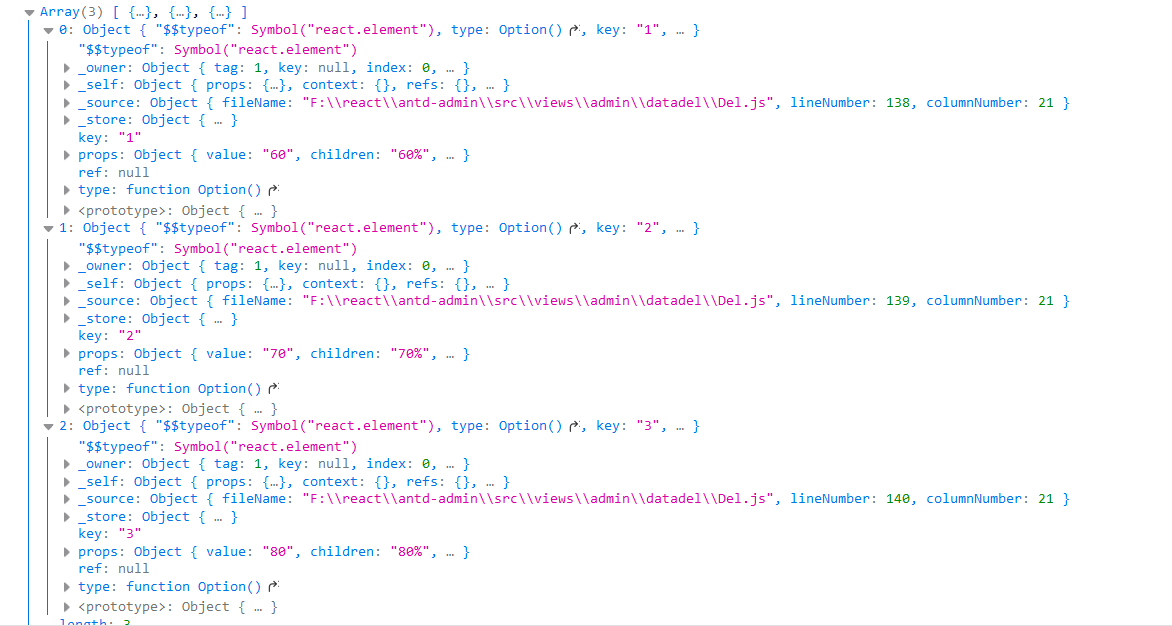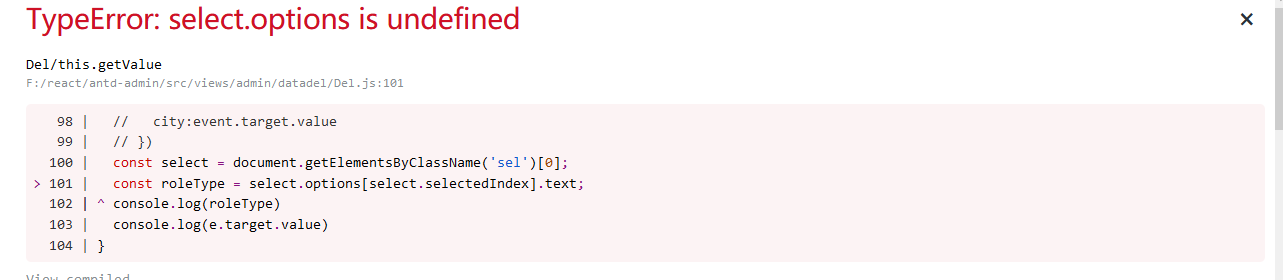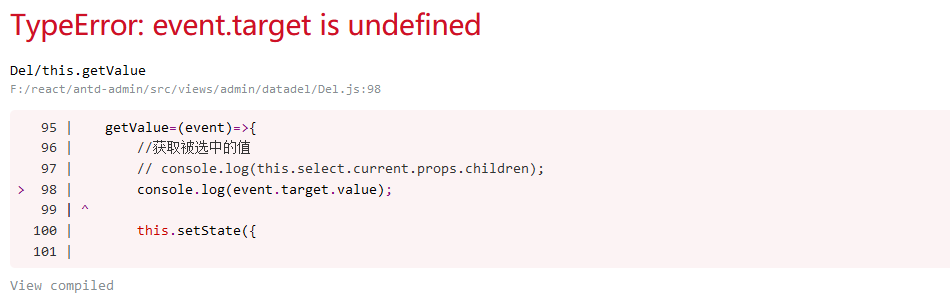2021-06-02 22:51

# react中如何获取select选中的值

``````<Select defaultValue="60%" ref={this.select} onChange={this.getValue} >
<Option key="1" value="60">60%</Option>
<Option key="2" value="70">70%</Option>
<Option key="3" value="80">80%</Option>
</Select>``````

``````getValue=(e)=>{
//获取被选中的值
console.log(this.select.current.props.children);

}````````````<Select defaultValue="60%" onChange={this.getValue} className="sel" >
<Option key="1" value="60">60%</Option>
<Option key="2" value="70">70%</Option>
<Option key="3" value="80">80%</Option>
</Select>``````

``````getValue=(e)=>{

const select = document.getElementsByClassName('sel');
const roleType = select.options[select.selectedIndex].text;
console.log(roleType)
}
``````• 点赞
• 写回答
• 关注问题
• 收藏
• 邀请回答

#### 3条回答默认 最新

•易书生 2021-06-02 23:10
已采纳
``````import React, { Component } from 'react'
class App extends Component {
constructor(props) {
super(props);
this.state = {
cities:["北京","上海","广州"],   //选项
city:"广州"   // 默认选项
}
}
render() {
return (
<div>
{/* value放在select里 select框也要onChcange*/}
<select value={this.state.city} onChange={(e)=>this.getValue(e)}>
{
// 遍历option
this.state.cities.map((ele,index)=>{
return(
<option key={index}>{ele}</option>
)
})
}
</select>
</div>
);
}
getValue=(event)=>{
//获取被选中的值
console.log(event.target.value);
this.setState({
//默认值改变
city:event.target.value
})

}
}

export default App;``````

可以试试这个

点赞 评论
•qq_46019299 2021-06-02 23:31我点击下拉框选择值之后，他报错了

点赞 评论
•你看看这个

``````import React, { Component, useState, useEffect } from 'react';
import { Tree } from 'antd';
import moment from "moment";
import Form from '../components/Form.jsx'

class Index extends Component{
change = (val) => {
console.log(val.target.value)
}
render(){
return (
<div>
<select onChange={this.change}>
<option value="1">222</option>
<option value="5">2</option>
</select>
</div>
)
}

}
export default Index``````
点赞 评论